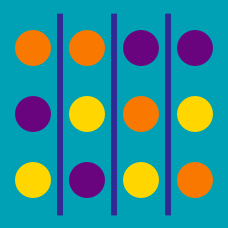Probability

Permutations with Repetition

The digits $1, 7,$ and $8,$ and $5$ copies of the digit $5$ are all arranged to form an 8-digit integer. How many different integers can be formed?

If $P^n_5 = 20 \cdot$ $P^n_3,$ what is the value of $n?$

Three flags colored yellow, red, and blue are prepared for sending signals. Each signal consists of one, two, or three flags where repetition in flag color is allowed. For example, $\text{red, yellow}$ and $\text{blue, blue, red}$ are two possible signals.

How many distinct signals can be made?

How many ways can three dogs and two cats be seated around a circular table? Consider two animals of the same type to be identical, and configurations that can be rotated to match are also considered identical.

Yan was opening a new restaurant, so she went to the sign store to get letters to make a sign to hang above the storefront. When she got to the sign store, the only letters they had in stock were two copies of the letter 'X,' two copies of the letter 'E,' and one copy of the letter 'V.' Yan decided to buy all the letters and use all of them to make the name of her store. How many different names can she give her store using these 5 letters?

Details and assumptions

The letters don't have to make a real word. For example, she can use the name XXVEE.

×